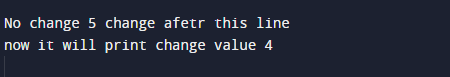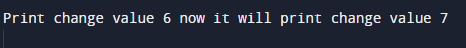# Increment Decrement Operators Program in Java

In this tutorial you will learn about the Increment Decrement Operators Program in Java and its application with practical example.

In this tutorial, we will learn to create a Increment Decrement Operators Program in Java using   java programming.

## Prerequisites

Before starting with this tutorial, we assume that you are best aware of the following Java programming concepts:

• Java Operators.
• Basic Input and Output function in Java.
• Class and Object in Java.
• Basic Java programming.
• If-else statements in Java.
• For loop in Java.

## Increment ++ and Decrement — Operator?

Operator “++” increases the value of a variable by 1 it means if value of a variable is “a=5” then “a++” gives as a=6;
Similarly, Decrement operator “–“ decreases the value of a variable by 1 it means  if “a=5” then “a–“ gives as a=4;
Both operators are unary operators. These operators  work on a single operand, that’s why they are called as unary operators.

## Increment Decrement Operators Program in Java

In this program we will define Increment Decrement Operators .We would first declared and initialized the required variables. Next, we will define Increment Decrement Operators . Lets have a look at the code

## Output## Output## Pre Increment operator ++

If we use “++” operator as  prefix  => ++var, then value of var is incremented by 1 then it returns  value.

## Output## Pre Decrement operator —

If we use “–“ operator as  prefix  => —var, then value of var is decremented by 1 then it returns  value.

## OutputIn this tutorial we have learn about the Increment Decrement Operators Program in Java and its application with practical example. I hope you will like this tutorial.